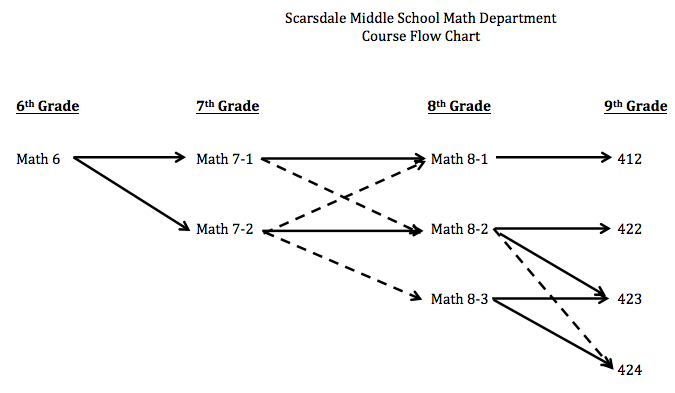Grade 6 classes are heterogeneously grouped.  The course covers the sixth grade curriculum and begins the seventh grade curriculum.

Students will:

• evaluate expressions using rational numbers (whole numbers, decimals, fractions, integers)

• apply properties of addition and multiplication in various problem solving situations

• solve one-step, two-step, and multi-step algebraic equations using the algebraic process with rational numbers

• use algebra in various problem solving situations

• explore basic number theory

• use visualization and spatial reasoning to analyze characteristics of geometric shapes and to calculate their area and volume

• be introduced to coordinate planes

• use ratio, proportion and percentages for problem solving and application

• be introduced to statistics and analysis with central tendencies

• engage in critical and creative thinking through various problem solving activities

Placement process for 7th grade math:
Placements for seventh grade are made by the sixth grade teacher and shared with parents via Infinite Campus in June.  There is one section of Math 7-1 and three sections of Math 7-2 in each house.  When making recommendations to the higher level math course (Math 7-2), teachers look for these attributes in their students:
• Displays self-advocacy skills (voluntarily seeking help and clarification when needed)
• Able to independently initiate and sustain working on math tasks
• Possesses an enjoyment and enthusiasm for the “challenges” of higher-level problem solving tasks
• Willingness to take mathematical risks
• Able to work collaboratively in groups to perform problem solving tasks
• Able to communicate mathematical thinking and understanding
• Exhibits resilience and a willingness to persist when faced with difficulty or struggle
• Can view problems from multiple perspectives with multiple ways to solve
• Displays reasoning capabilities that have shifted from a concrete to an abstract nature**Solid lines indicate a typical path.  Dashed lines indicate possible paths that usually occur with intermediate steps taken.